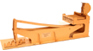The ternary calculating machine of Thomas Fowler home - about - contact Thomas Fowler Balanced ternary arithmetic Fowler's binary and ternary tables DeMorgan's description The reconstruction About the machine Overview Multiplicand Multiplier Product Carry mechanism Instructions for use Video demonstration Photo gallery References and links Acknowledgements Wanted Fowler's ternary calculating machine - instructions for use These are the steps required to calculate a multiplication problem using Fowler's machine. 1) Reset all the product rods to zero. 2) Enter each digit of the multiplicand by sliding the individual multiplicand rods into position. 3) Enter each digit of the multiplier by clipping the ends of the multiplier rods onto the appropriate rung of the ladder-like rotating frame. 4) Move the rotating frame on the multiplier to the vertical (neutral) position. 5) Slide the entire multiplier mechanism sideways to engage the tooth on the rotating frame of the multiplier into the slot of the first multiplicand rod. 6) Rotate the frame in the direction that would move the multiplicand rod to the zero position. 7) Slide the entire multiplier assembly sideways to an intermediate position, so that the tooth on the rotating frame disengages from the first multiplicand rod and ends up in the space between two multiplicand rods. 8) Repeat steps 4 through 7 for each digit of the multiplicand. 9) At the end of the calculation, or during the calculation if any of the product rods have an overflow of three units, engage the carry mechanism to eliminate any overflows.